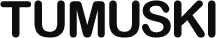Pages: 1 2 3 4 5 6

# Clumpy Usage Guide

## Clumpy Has Moved

It’s here now: https://thomasperi.github.io/clumpyjs/

First things first:

``````<script type="text/javascript" src="Clumpy.js"></script>
<script type="text/javascript">
var clumpy = new Clumpy();
</script>``````

Now onto the good stuff. As you may remember from the overview, here’s how to model a `for` loop in Clumpy:

``````var i;
clumpy.for_loop(
function () { i = 0; },
function () { return i < 100000; },
function () { i++; },
function () {
// statements
}
);``````

It looks a little strange, being so heavy on the functions, but you can see the resemblance it bears to a real `for` loop. A notable difference, though, is that the second function returns the result of the condition, where there is no return statement in a real `for` loop.

## Reinventing the “While”

The methods that model `while` and `do...while` are similar to `for_loop()`:

``````var i = 0;
clumpy.while_loop(
function () { return i < 100000; },
function () {
// statements
}
);``````
``````var i = 0;
clumpy.do_while_loop(
function () {
// statements
},
function () { return i < 100000; }
);``````

`for...in` loops are almost as straightforward:

``````var key, object = { ... };

// Real Loop
for (key in object) {
// statements
}

// Loose Equivalent:
clumpy.for_in_loop(
function () { return object; },
function (key) {
// statements
}
);

// Strict Equivalent:
clumpy.for_in_loop(
function () { return object; },
function (k) {
key = k;
// statements
}
);``````

The difference between the “loose” and “strict” equivalents is that the `key` parameter to the function is not the same `key` variable that’s declared outside the function. The strict example makes the value persist in the outer `key`.

Next Page: Chains